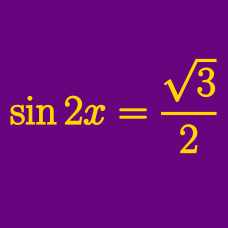Geometry

# Trigonometric Equations - Sum to Product

Which of the following is equal to $8\cos x-8\cos (x-60^\circ) ?$

What is the value of $\sin {56}^\circ-\sin {64}^\circ+\sin {176}^\circ ?$

If $$\displaystyle x=\frac{\pi}{15}$$ what is the value of $\frac{\cos x \cos 7x}{\cos 9x + \cos 7x} ?$

If $$\displaystyle x=\frac{\pi}{96}$$ what is the value of $\frac{\cos 14x + \cos 16x + \cos 18x}{\sin 14x + \sin 16x + \sin 18x} ?$

Which of the following expressions is equal to $7\sqrt{3}\sin \left(\theta+\frac{\pi}{3}\right)-14\cos \theta ?$

×

Problem Loading...

Note Loading...

Set Loading...